Test: Differential Calculus

# Test: Differential Calculus

Test Description

## 20 Questions MCQ Test GATE Electrical Engineering (EE) 2023 Mock Test Series | Test: Differential Calculus

Test: Differential Calculus for Electronics and Communication Engineering (ECE) 2023 is part of GATE Electrical Engineering (EE) 2023 Mock Test Series preparation. The Test: Differential Calculus questions and answers have been prepared according to the Electronics and Communication Engineering (ECE) exam syllabus.The Test: Differential Calculus MCQs are made for Electronics and Communication Engineering (ECE) 2023 Exam. Find important definitions, questions, notes, meanings, examples, exercises, MCQs and online tests for Test: Differential Calculus below.
Solutions of Test: Differential Calculus questions in English are available as part of our GATE Electrical Engineering (EE) 2023 Mock Test Series for Electronics and Communication Engineering (ECE) & Test: Differential Calculus solutions in Hindi for GATE Electrical Engineering (EE) 2023 Mock Test Series course. Download more important topics, notes, lectures and mock test series for Electronics and Communication Engineering (ECE) Exam by signing up for free. Attempt Test: Differential Calculus | 20 questions in 60 minutes | Mock test for Electronics and Communication Engineering (ECE) preparation | Free important questions MCQ to study GATE Electrical Engineering (EE) 2023 Mock Test Series for Electronics and Communication Engineering (ECE) Exam | Download free PDF with solutions
 1 Crore+ students have signed up on EduRev. Have you?
Test: Differential Calculus - Question 1

### If u = xm yn  then

Detailed Solution for Test: Differential Calculus - Question 1

Given that u = xyn

Taking logarithm of both sides, we get log u = m log x + n log y Differentiating with respect to x,we get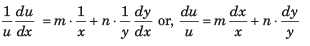Test: Differential Calculus - Question 2

###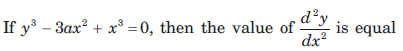​ ​ ​  to

Detailed Solution for Test: Differential Calculus - Question 2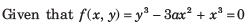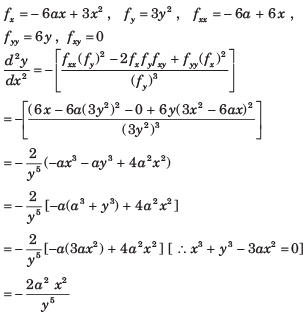Test: Differential Calculus - Question 3

###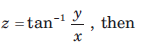​ ​ ​

Detailed Solution for Test: Differential Calculus - Question 3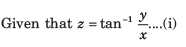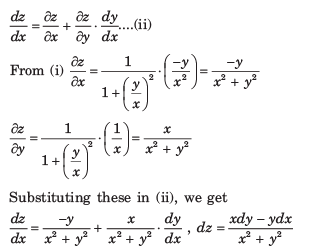Test: Differential Calculus - Question 4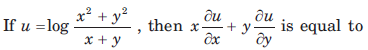Detailed Solution for Test: Differential Calculus - Question 4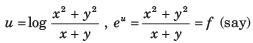f is a homogeneous function of degree one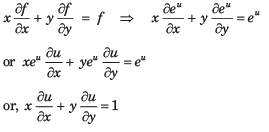Test: Differential Calculus - Question 5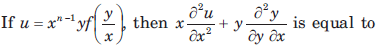Detailed Solution for Test: Differential Calculus - Question 5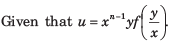It is a homogeneous function of degree n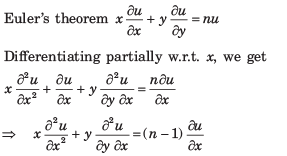Test: Differential Calculus - Question 6

Match the List–I with List–II.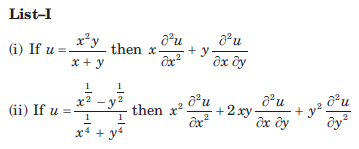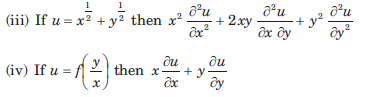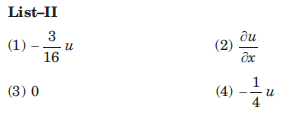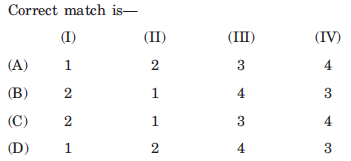Detailed Solution for Test: Differential Calculus - Question 6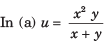It is a homogeneous function of
degree 2.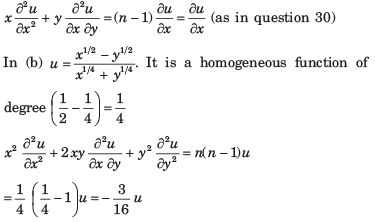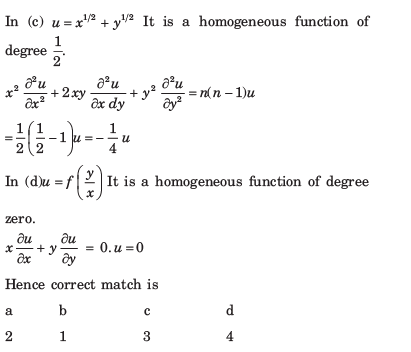Test: Differential Calculus - Question 7

If an error of 1% is made in measuring the major and minor axes of an ellipse, then the percentage error in the area is approximately equal to

Detailed Solution for Test: Differential Calculus - Question 7

Let 2 a and 2 b be the major and minor axes of the ellipse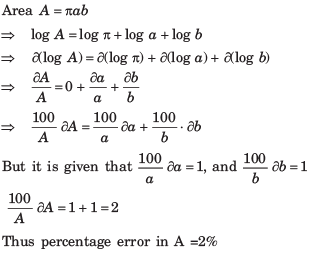Test: Differential Calculus - Question 8

Consider the Assertion (A) and Reason (R) given below: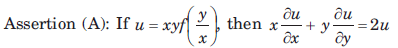Reason (R): Given function u is homogeneous of degree 2 in x and y.
Of these statements

Detailed Solution for Test: Differential Calculus - Question 8

Given that  u = xyf(y/x) Since it is a homogeneous function of degree 2.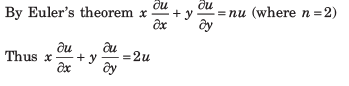Test: Differential Calculus - Question 9

If u = x log xy, where x3 + y3 + 3xy = 1, then du/dx is equal to

Detailed Solution for Test: Differential Calculus - Question 9

Given that u = x log xy ... (i)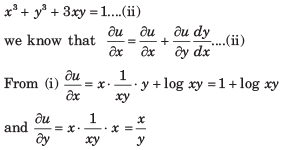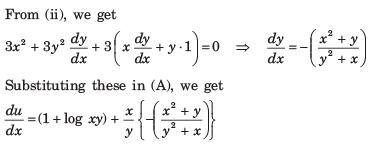Test: Differential Calculus - Question 10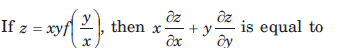Detailed Solution for Test: Differential Calculus - Question 10

The given function is homogeneous of degree 2.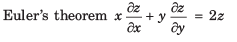Test: Differential Calculus - Question 11

If a < 0, then f(x) = eax + e-ax is decreasing for

Detailed Solution for Test: Differential Calculus - Question 11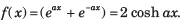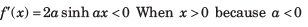Test: Differential Calculus - Question 12

f(x) = x2e-x is increasing in the interval

Detailed Solution for Test: Differential Calculus - Question 12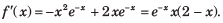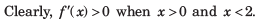Test: Differential Calculus - Question 13

The least value of a for which f(x) = x2 + ax + 1 is increasing on ] 1, 2, [ is

Detailed Solution for Test: Differential Calculus - Question 13

f'(x) = (2x + a)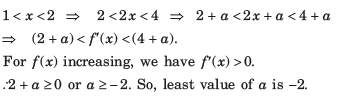Test: Differential Calculus - Question 14

The minimum distance from the point (4, 2) to the parabola y2 =​ 8x is

Detailed Solution for Test: Differential Calculus - Question 14

Let the point closest to (4, 2) be (2t2,4)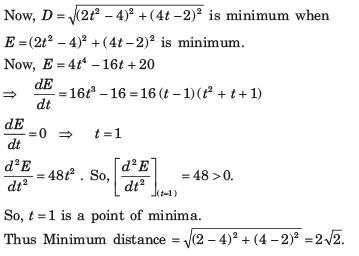Test: Differential Calculus - Question 15

The co-ordinates of the point on the parabola y = x2 + 7x + 2 which is closest to the straight line y = 3x - 3, are

Detailed Solution for Test: Differential Calculus - Question 15

Let the required point be P(x, y). Then, perpendicular distance of P(x, y) from y -  3x - 3 = 0 is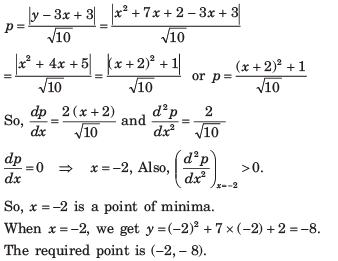Test: Differential Calculus - Question 16

The shortest distance of the point (0, c), where 0 ≤ c ≤ 5, from the parabola y = x2 is

Detailed Solution for Test: Differential Calculus - Question 16

Let A (0,c) be the given point and P (x, y) be any point on y = x2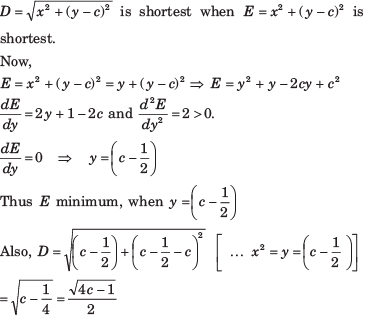Test: Differential Calculus - Question 17

The maximum value of ( 1/x)x is

Detailed Solution for Test: Differential Calculus - Question 17

f (x) = (1 / x)x

f’ (x) = (1 / x)x (log (1 / x) – 1))

f’ (x) = 0

log (1 / x) – 1 = log e

1 / x = e

x = 1 / e

The maximum value of function is e1/e.

Test: Differential Calculus - Question 18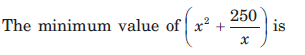Detailed Solution for Test: Differential Calculus - Question 18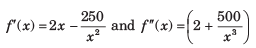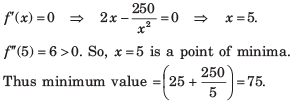Test: Differential Calculus - Question 19

The maximum value of f ( x) = (1 + cos x) sin x is

Detailed Solution for Test: Differential Calculus - Question 19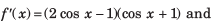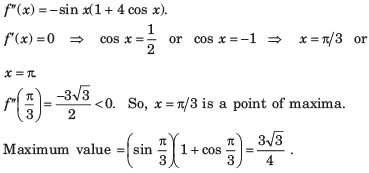Test: Differential Calculus - Question 20

The greatest value of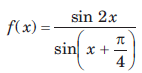on the interval [0, π/2] is

Detailed Solution for Test: Differential Calculus - Question 20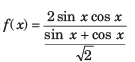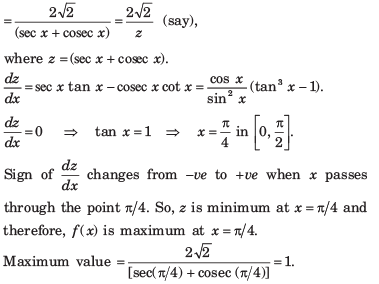## GATE Electrical Engineering (EE) 2023 Mock Test Series

22 docs|274 tests
 Use Code STAYHOME200 and get INR 200 additional OFF Use Coupon Code
Information about Test: Differential Calculus Page
In this test you can find the Exam questions for Test: Differential Calculus solved & explained in the simplest way possible. Besides giving Questions and answers for Test: Differential Calculus, EduRev gives you an ample number of Online tests for practice

## GATE Electrical Engineering (EE) 2023 Mock Test Series

22 docs|274 tests